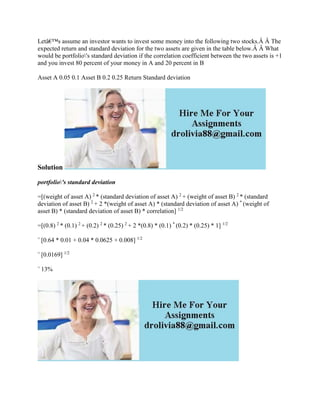Se está descargando tu SlideShare. ×

# Lets assume an investor wants to invest some money into the following.docx

Anuncio
Anuncio
Anuncio
Anuncio
Anuncio
Anuncio
Anuncio
Anuncio
Anuncio
Anuncio
AnuncioCargando en…3
×

1 de 1 Anuncio

# Lets assume an investor wants to invest some money into the following.docx

Letâ€™s assume an investor wants to invest some money into the following two stocks.Â Â The expected return and standard deviation for the two assets are given in the table below.Â Â What would be portfolio\'s standard deviation if the correlation coefficient between the two assets is +1 and you invest 80 percent of your money in A and 20 percent in B
Asset A 0.05 0.1 Asset B 0.2 0.25 Return Standard deviation
Solution
portfolio\'s standard deviation
=[(weight of asset A) 2 * (standard deviation of asset A) 2 + (weight of asset B) 2 * (standard deviation of asset B) 2 + 2 *(weight of asset A) * (standard deviation of asset A) * (weight of asset B) * (standard deviation of asset B) * correlation] 1/2
=[(0.8) 2 * (0.1) 2 + (0.2) 2 * (0.25) 2 + 2 *(0.8) * (0.1) * (0.2) * (0.25) * 1] 1/2
= [0.64 * 0.01 + 0.04 * 0.0625 + 0.008] 1/2
= [0.0169] 1/2
= 13%
.

Letâ€™s assume an investor wants to invest some money into the following two stocks.Â Â The expected return and standard deviation for the two assets are given in the table below.Â Â What would be portfolio\'s standard deviation if the correlation coefficient between the two assets is +1 and you invest 80 percent of your money in A and 20 percent in B
Asset A 0.05 0.1 Asset B 0.2 0.25 Return Standard deviation
Solution
portfolio\'s standard deviation
=[(weight of asset A) 2 * (standard deviation of asset A) 2 + (weight of asset B) 2 * (standard deviation of asset B) 2 + 2 *(weight of asset A) * (standard deviation of asset A) * (weight of asset B) * (standard deviation of asset B) * correlation] 1/2
=[(0.8) 2 * (0.1) 2 + (0.2) 2 * (0.25) 2 + 2 *(0.8) * (0.1) * (0.2) * (0.25) * 1] 1/2
= [0.64 * 0.01 + 0.04 * 0.0625 + 0.008] 1/2
= [0.0169] 1/2
= 13%
.

Anuncio
Anuncio

### Lets assume an investor wants to invest some money into the following.docx

1. 1. Letâ€™s assume an investor wants to invest some money into the following two stocks.Â Â The expected return and standard deviation for the two assets are given in the table below.Â Â What would be portfolio's standard deviation if the correlation coefficient between the two assets is +1 and you invest 80 percent of your money in A and 20 percent in B Asset A 0.05 0.1 Asset B 0.2 0.25 Return Standard deviation Solution portfolio's standard deviation =[(weight of asset A) 2 * (standard deviation of asset A) 2 + (weight of asset B) 2 * (standard deviation of asset B) 2 + 2 *(weight of asset A) * (standard deviation of asset A) * (weight of asset B) * (standard deviation of asset B) * correlation] 1/2 =[(0.8) 2 * (0.1) 2 + (0.2) 2 * (0.25) 2 + 2 *(0.8) * (0.1) * (0.2) * (0.25) * 1] 1/2 = [0.64 * 0.01 + 0.04 * 0.0625 + 0.008] 1/2 = [0.0169] 1/2 = 13%